# 5.5 WeightPosition Interpolations

Different interpolation types allow inbetweens to be blended in and out in a smooth way. Take the example of two weightPositions of a weight, one at 0.5 (`wp1`) and one at 1 (`wp2`). With linear interpolation, the WPs are weighted according to the following plot: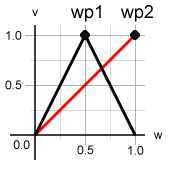Now consider a (trivial) plain dataPoint that simply moves a vertical bar down, like this:

No Inbetween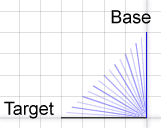Adding an inbetween to get a more arced movement results in the following motion in case linear interpolation is used:

Linear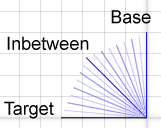Here are two examples where the WP at 0.5 (`wp1`) has been set to a non-linear interpolation:

SplineArc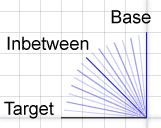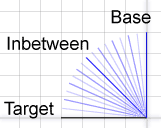There are other interpolations, too. They are described in the Glossary under WPos Interpolation.